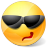# Math Is Fun Forum

Discussion about math, puzzles, games and fun.   Useful symbols: ÷ × ½ √ ∞ ≠ ≤ ≥ ≈ ⇒ ± ∈ Δ θ ∴ ∑ ∫  π  -¹ ² ³ °

You are not logged in.

## #1 2014-07-31 22:53:11

careless25
Real MemberRegistered: 2008-07-24
Posts: 560

### Probability Random Variable with Central Limit Theorem

At a bank, the interest is calculated on 100 accounts and rounded up or down
to nearest cent. If it is assumed that the round-off error is uniformly distributed between
(-1/2, 1/2) and
the round-off errors are independent, find the probability that the sum
of the error does not exceed 2 cents in magnitude.

I dont understand how to calculate the variance for just one account. the answer for variance for just one account is 1/12.

Offline

## #2 2014-08-01 20:53:02

bobbym
bumpkinFrom: Bumpkinland
Registered: 2009-04-12
Posts: 109,606

### Re: Probability Random Variable with Central Limit Theorem

Hi;

I am getting:

In mathematics, you don't understand things. You just get used to them.
If it ain't broke, fix it until it is.
Always satisfy the Prime Directive of getting the right answer above all else.

Offline

## #3 2014-08-02 17:44:21

careless25
Real MemberRegistered: 2008-07-24
Posts: 560

### Re: Probability Random Variable with Central Limit Theorem

Hi bobbym,

Yes that is the correct answer. I was asking about calculating the variance not the probability.

Var(X) = E(x^2) - E(x)^2

and f(x) = 1 as this is uniform distribution from -1/2 to 1/2.

so:

Using Central Limit Theorem:

is the square root of the variance calculated above.
is the standard distribution.

Therefore P(-2 <= x <= 2) = 0.512

Last edited by careless25 (2014-08-02 18:26:22)

Offline